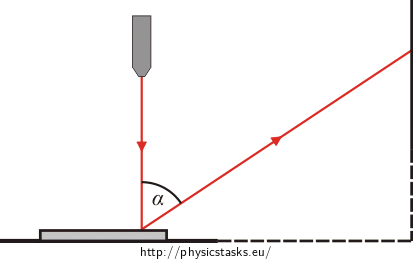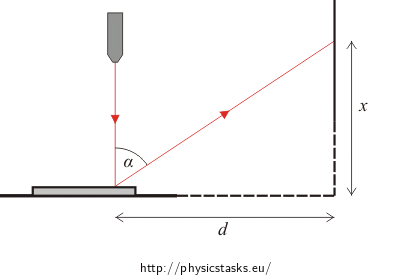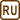## DVD as an Optical Grating

Edward, Eugene and Eve attended a laboratory lesson at school. They placed a DVD on a flat surface and pointed a laser with a wavelength of 650 nm to the DVD so that the ray was perpendicular to the DVD. First-order maxima appeared on a wall at a distance of 130 cm from the DVD and at a height of 70 cm. The figure below shows the arrangement of this experiment.

a) What is the distance between two adjacent recording tracks on the DVD?

b) How many tracks are there on 1 mm of length?• #### Hint 1

A DVD behaves like an optical grating. Do you know the relationship which applies for the path difference of rays?

• #### Hint 2

Determine the deflection angle α of the first maximum using given distances.

• #### Given values

 d = 130 cm = 1.3 m Distance between the DVD and the wall k = 1 Order of maximum λ = 650 nm = 6.5·10−7 m Wavelength of light x = 70 cm = 0.7 m Height of first-order maximum b = ? (nm) Distance between two tracks on a DVD N = ? Number of tracks per 1 mm of length
• #### Solution

In this task, the DVD acts as an optical grating which reflects light. This grating behaves almost like a grating through which the light passes. The only difference is that the diffraction phenomena occur on the same side of the grating where the light source is.

In this task we want to determine the distance of two tracks and how many tracks is there per 1 mm of length. For this calculation we need to know the relation for the deflection angle αand the relationship for the path difference Δl of the rays.From the picture we obtain a relationship:

$\mathrm{tg}\,\,α=\,\frac{d}{x},$

where d is the distance between the DVD and the wall and x is the height at which the first-order maxima appear.

For the path difference Δl the following applies:

$\mathrm{Δ}l = \,b\sin\,α,$

where Δl is the path difference of the rays, b is the distance between two tracks on the DVD and α is the deflection angle.

For Δl we substitute a condition for a maximum Δl = , where k = 1.

We evaluate the distance b:

$b=\,\frac{\mathrm{Δ}l}{\sin\,α}=\,\frac{λ}{\sin\,α}.$

Numerical substitution

First, we calculate the angle using the equation:

$\mathrm{tg}\,\,α=\,\frac{d}{x}=\,\frac{1.3}{0.7}\,\dot=\,1.86 \quad\Rightarrow\quad α\,\dot=\,61.74°$

and then we calculate the distance b between two tracks

$b=\,\frac{6.5{\cdot}10^{−7}}{\sin\,61.74}\,\mathrm{m}\,\dot=\,7.40{\cdot}10^{−7}\,\mathrm{m}\,\dot=\,740\,\mathrm{nm}.$

Number of tracks N per 1 mm can be obtained as follows:

$N=\,\frac{10^{−3}\,\mathrm{m}}{b}=\,\frac{10^{−3}\,\mathrm{m}}{7.38{\cdot}10^{−7}}\,\dot=\,1400.$